# Height

The content of the triangle is 35 cm2. The length of the base is 10 cm. Determine the length of the height on the base.

v =  7 cm

### Step-by-step explanation: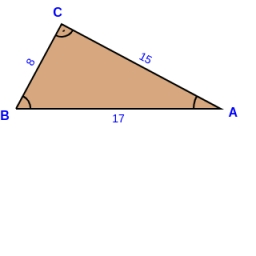Did you find an error or inaccuracy? Feel free to write us. Thank you!Tips to related online calculators

#### You need to know the following knowledge to solve this word math problem:

We encourage you to watch this tutorial video on this math problem:

## Related math problems and questions:

• Height to the baseThe triangle area is 35 cm ^ 2. The size of the base is 10 cm. Find the length of height to the base.
• 7 triangle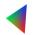The triangle area is 26.7 cm2. Determine the side length l if appropriate height hl = 45.3 cm.
• Rectangular trapeziumCalculate the perimeter of a rectangular trapezium when its content area is 576 cm2 and sice a (base) is 30 cm, height 24 cm.
• Parallerogram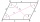In parallelogram ABCD is a = 2.5 cm and height to side a is 7.2 cm and height to side b is 4 cm. Find the side length b.
• Perimeter from areaWhat is the perimeter of the square if its content is 64 cm2?
• Cube 5The content area of one cube wall is 32 square centimeters. Determine the length of its edges, its surface and volume.
• The perimeter 3The perimeter of a rectangle is 35 cm. The ratio of the length to its width is 3:2. Calculate the dimensions of the rectangle
• Calculate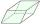Calculate the content cm2 diamond with side length 9.8 cm and a height on the side of 5 cm.
• Four prismsQuestion No. 1: The prism has the dimensions a = 2.5 cm, b = 100 mm, c = 12 cm. What is its volume? a) 3000 cm2 b) 300 cm2 c) 3000 cm3 d) 300 cm3 Question No.2: The prism base is a rhombus with a side length of 30 cm and a height of 27 cm. The height of t
• Arm-legCalculate the length of the base of an isosceles triangle with a circumference 224 cm if the arm length is 68 cm.
• Cuboid surfaceDetermine surface area of cuboid if its volume is 52.8 cm cubic and length of the two edges are 2 cm and 6 cm.
• 2d shapeCalculate the content of a shape in which an arbitrary point is not more than 3 cm from the segment AB. The length of the segment AB is 5 cm.
• TrapeziumDetermine the height of the trapezium ABCD, which has area 77.5 cm2 and base lengths 16 cm and 15 cm.
• IS trapezoid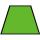Isosceles trapezoid arm measured 35 cm. Height is 30 cm and middle segment is 65 cm. Determine length of its bases.
• Perimeter to area 2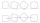Find the area of a square whose perimeter is 100 cm with solve.
• Rectangle 45The perimeter of a rectangle is 60cm. If the length of the rectangle is 20cm. a)find the width b)find the area.
• An equilateral triangle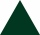The perimeter of an equilateral triangle is 33cm. How long is each side?